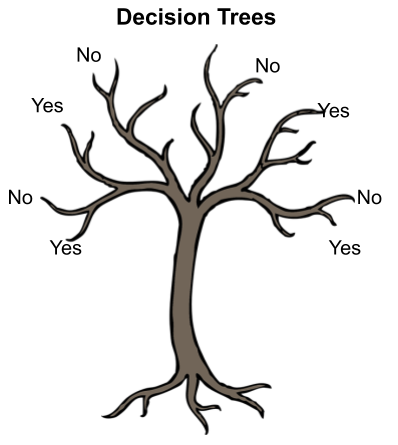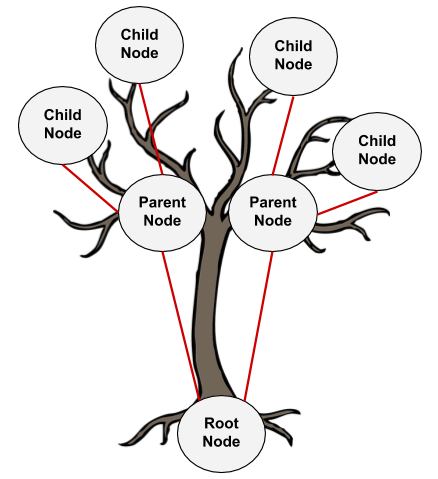# Decision Tree IntuitionDecision Tree Intuition. In Programming, we regularly use If-else conditions, even the Decision Tree working process is similar to an If-else condition.

Decision Trees are easy & Simple to implement & interpreted. Decision Tree is a diagram (flow) that is used to predict the course of action or a probability. Each branch of the decision tree represents an outcome or decision or a reaction. Decision Trees can be implemented in a variety of situations from personal to complex situations. The sequence of steps will give a better understanding easily.

In Programming, we regularly use If-else conditions, even the Decision Tree working process is similar to an If-else condition.

## Let’s see how a decision Tree Looks like

The below tree shows a simple implementation of different nodes in the decision tree.1. *Root Node: *Root Node is a top node with the base feature.
2. *Parent Node: *Nodes that get their origin from a root node or this can be represented as a decision node where the decision of Yes/No or True/False or prediction turn happens.
3. *Child Node: *these nodes get their origin from a parent node. If the decision made from a parent node is not satisfactory then these nodes will be created. Until we arrive at the final node where we have pure domination in a class (Yes/No) means that until we arrive at the leaf node.
4. *Leaf Node: *Can also be called a terminal Node or a final decision node where we will conclude.

## Decision Tree -Classification

Decision Tree is one of the most widely used machine learning algorithm. It is a supervised learning algorithm that can perform both classification and regression operations.

## Decision Trees Classifier

Decision Trees Classifier - Both of Regression Trees and Classification Trees are a part of CART (Classification And Regression Tree) Algorithm.

## Maths Behind Decision Tree Classifier

Maths behind Decision Tree Classifier. Before we see the python implementation of the decision tree. Let’s first understand the math behind the decision tree classification.

## Simplifying the Decision Tree in Machine Learning

One of the most popular and used ML Algorithm. It’s one of the most simple and basic models of machine learning, which can be used both for classification and regression.

## Machine Learning: Decision Trees

Machine Learning: Decision Trees. This blog covers another interesting machine learning algorithm called Decision Trees and it’s mathematical implementation.